# Bootstrapping in R: Parametric vs Nonparametric BootstrapWe first need to understand following some fundamental concepts. The idea behind bootstrapping is very closely aligned with those concepts.

Here I have created a hypothetical study in which we are interested in finding out the average birth weight of the babies born in the UK at 37 weeks of gestation.

• Experiment : To find out the average weight of babies born at 37 weeks in the UK

• Random sample : Random sample means each and every single individual or object has an equal probability of being chosen from the population. For example, in our study, the population is all birth happened in the UK at 37 weeks of gestation. For typical “random sample”, we will need to make sure that each and every birth happening in any corner of the UK, will be participating in our study, from which will choose some of them randomly i.e random sample.

• Sample data: the recorded observation or quantity from the sample of the population. Now we have gone to one local hospital and got data of the birth-weights from 20 babies. Here is the (hypothetical) sample data.

##   3331.608 3549.913 2809.252 2671.465 3383.177 3945.721 3672.601
##   3922.416 4647.278 4088.246 3718.874 3724.443 3925.457 3112.920
##  3495.621 3651.779 3240.194 3867.347 3431.015 4163.725
• Sample statistic : is the quantity of interest from sample data. The quantity of interest in our study is a mean (average). So mean is our sample statistic. So sample statistic in our sample data is 3618,
##  3617.653
• Sampling distribution (of statistic) : Now we went to take a random sample of 20 babies at numerous hospitals (i.e.multiple times) in the UK. Each time, we calculate the mean birth weight and note down its value. This value will be different in each different hospital (in a statistical sense, the statistic is a random variable, will vary as variation is inherent). Here we went to 100 hospitals and took a sample of 20 babies born at that hospital and calculated the mean birth weight and plotted in the histogram.
require(ggplot2)
ggplot(data.frame(statistic), aes(statistic)) + geom_histogram() + labs(x= "Mean", y= "Freqency")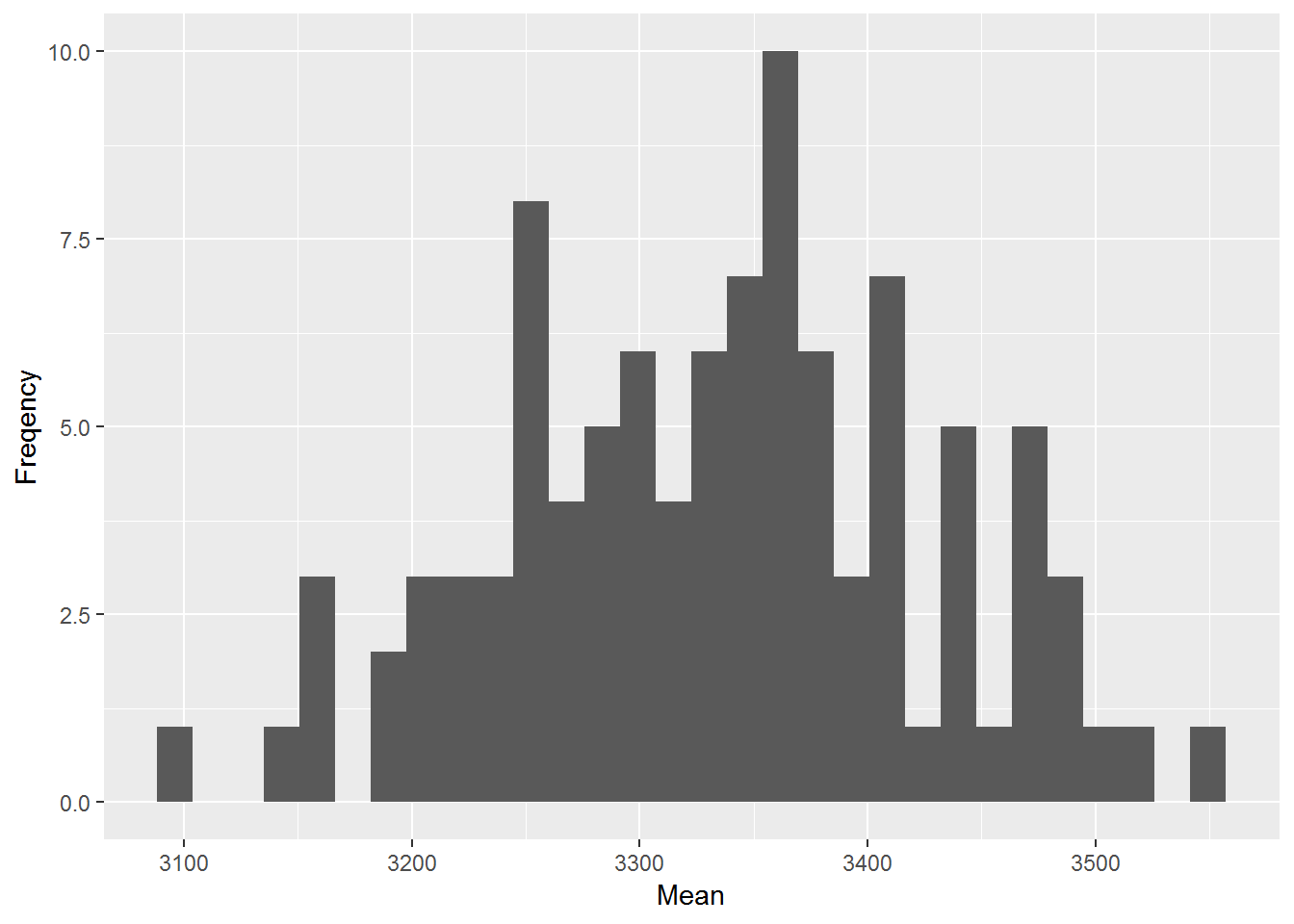Figure 1: Distribution of sample means

binw <- 2*(IQR(statistic)/length(statistic)^(1/3))
ggplot(data.frame(statistic), aes(statistic)) + geom_histogram(aes(y=..density..),binwidth = binw,fill="darkred",colour="black",size=1) + geom_density(aes(y=..density..),size=1) + labs(x= "Mean", y= "Density")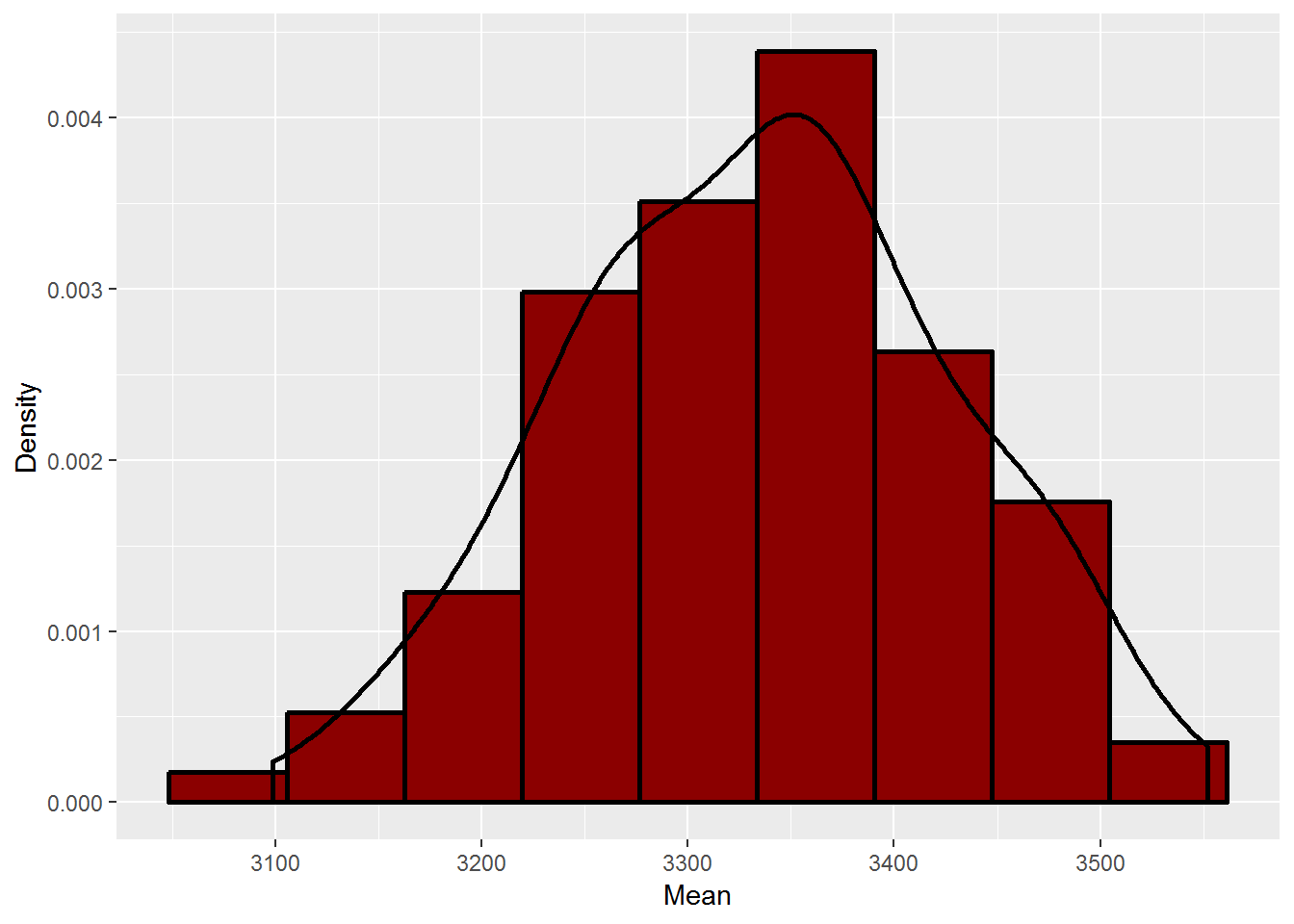Figure 2: Distribution of sample means (proper histogram)

• Population parameter : What will be the mean birth-weight of babies born in the UK if we had gone on collecting birthright of each and every single baby born in the UK. This will be only one value that what we are basically interested in. This is called the population parameter. The problem is that we want to estimate that from our limited number of observations (i.e sample data). The sample statistic will help to estimate the population parameter. The sample statistic will also be called point estimator.

• Standard error(of statistic) is the standard deviation (SD) of statistic from its sampling distribution. Note that SD is a statistic that measures the variability in data relative to its mean.

• Confidence interval : Though we are interested in population parameter i.e single value, what if our sample data may not contain it. We need to provide some measure of it. This can be achieved by building a confidence interval. We can say that the true value of the population lies in some interval with some degree of confidence.

So, in essence, we want to estimate a population parameter from the sampling distribution of the sample statistic. This sampling distribution can be generated by infinite time random sampling.

## Bootstrap concept

The bootstrap method is one type of re-sampling method, in which sample data (20 birth weights) considered as “population”.From this sample data, we re-sample it with a replacement-large number of time (the 1000’s). The resultant sampling distribution often (not always) approximate the (true) sampling distribution of the statistic.

Please note, that this sampling will be done with the replacement. So some single observation may be included many times ( or some may be left out completely). So sample statistic will be varied from each random sample of size n.

From this bootstrapped sampling distribution, which can estimate parameter value, standard errors (standard deviation of sample statistic) and then, calculate the confidence interval.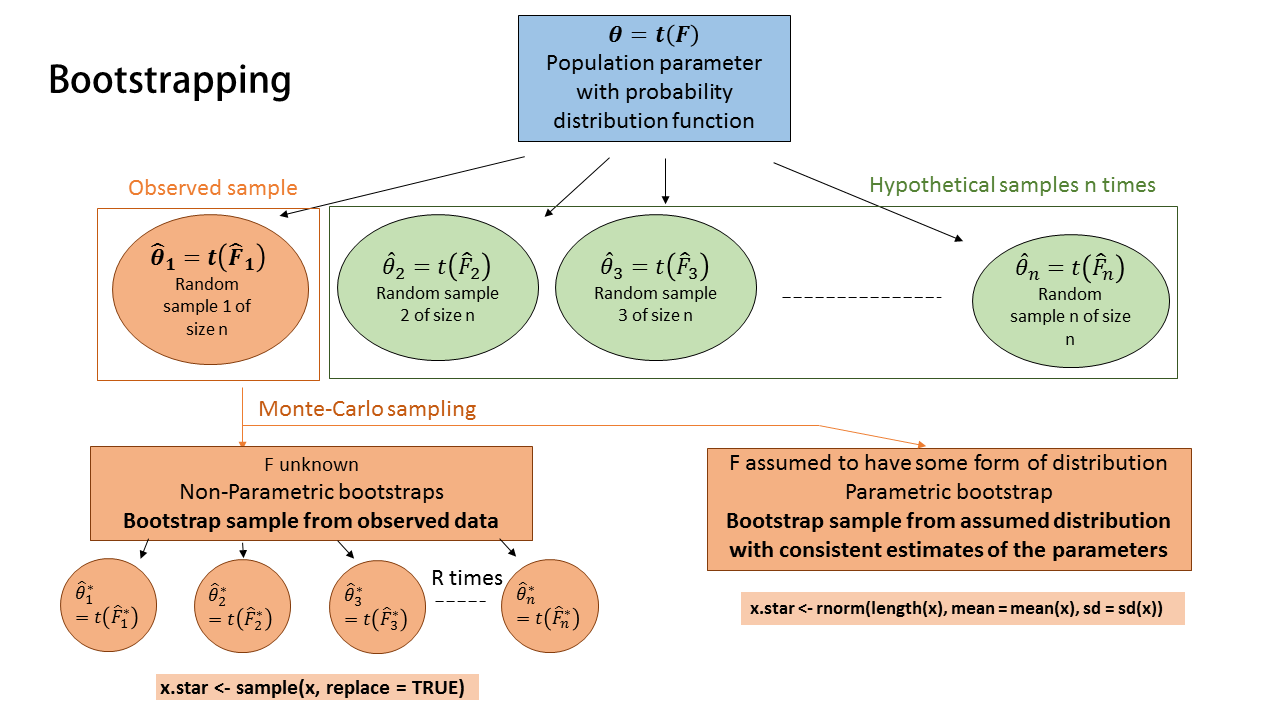Figure 3: Parametric vs nonparametric bootstrap

Let,

• $$\theta$$ is population parameter of interest which belongs to unknown population distribution F

• $$\hat{\theta}$$ is statistic that estimate the $$\theta$$ and we are interests in sampling distribution of $$\hat{\theta}$$ from fitted distribution function $$\hat{F}$$

• $$\hat{\theta_0^*}$$,$$\hat{\theta_1^*}$$,$$\hat{\theta_2^*}$$… bootstrap estimate (statistic) to obtain sampling distribution of $$\hat{\theta^*}$$ is $$\hat{F^*}$$. This sampling distribution is good approximation of $$\hat{F}$$

## Nonparametric bootstrap

We observed the sample data and we are unable to determine from which probability distribution function may have generated this sample data. In this situation, we use empirical distribution function (which is based on observed sample data) to simulate bootstrap samples (using Monte Carlo techniques).

# loop
theta_star <- vector()
for (i in 1:1000){
theta_star[i] <- mean(sample(df,size=length(df),replace = T))
# First take sample with replacement from obseerved data of same size & then, calculte sample statistic in each, repeat this 1000 times
}
theta_boot <- mean(theta_star) # bootstrap estimate of theta_hat
theta_boot
##  3616.166
boot_se <- sd(theta_star) # standard eorrs of the estimate
boot_se
##  102.6719
bias <- theta_boot - mean(df)
bias
##  -1.487018
MSE <- mean((theta_boot- mean(df))^2)
MSE
##  2.211222
CI <-c(theta_boot-1.96*boot_se,theta_boot +1.96*boot_se)
CI
##  3414.929 3817.402

We can use a boot function from the boot package in R.It requires a function to calculate sample statistic ( in its statistic argument). The function must include observed data as the first argument and indices ( or weight) in the second argument.

require(boot)
theta_star_function <- function(x,i) mean(x[i])
#
B <- boot(data = df, statistic = theta_star_function, R=1000, stype = "i" )
B
##
## ORDINARY NONPARAMETRIC BOOTSTRAP
##
##
## Call:
## boot(data = df, statistic = theta_star_function, R = 1000, stype = "i")
##
##
## Bootstrap Statistics :
##     original    bias    std. error
## t1* 3617.653 0.9443187    98.78257

We can used default plots to see whether bootstrap samples are correctly sampled.

plot(B)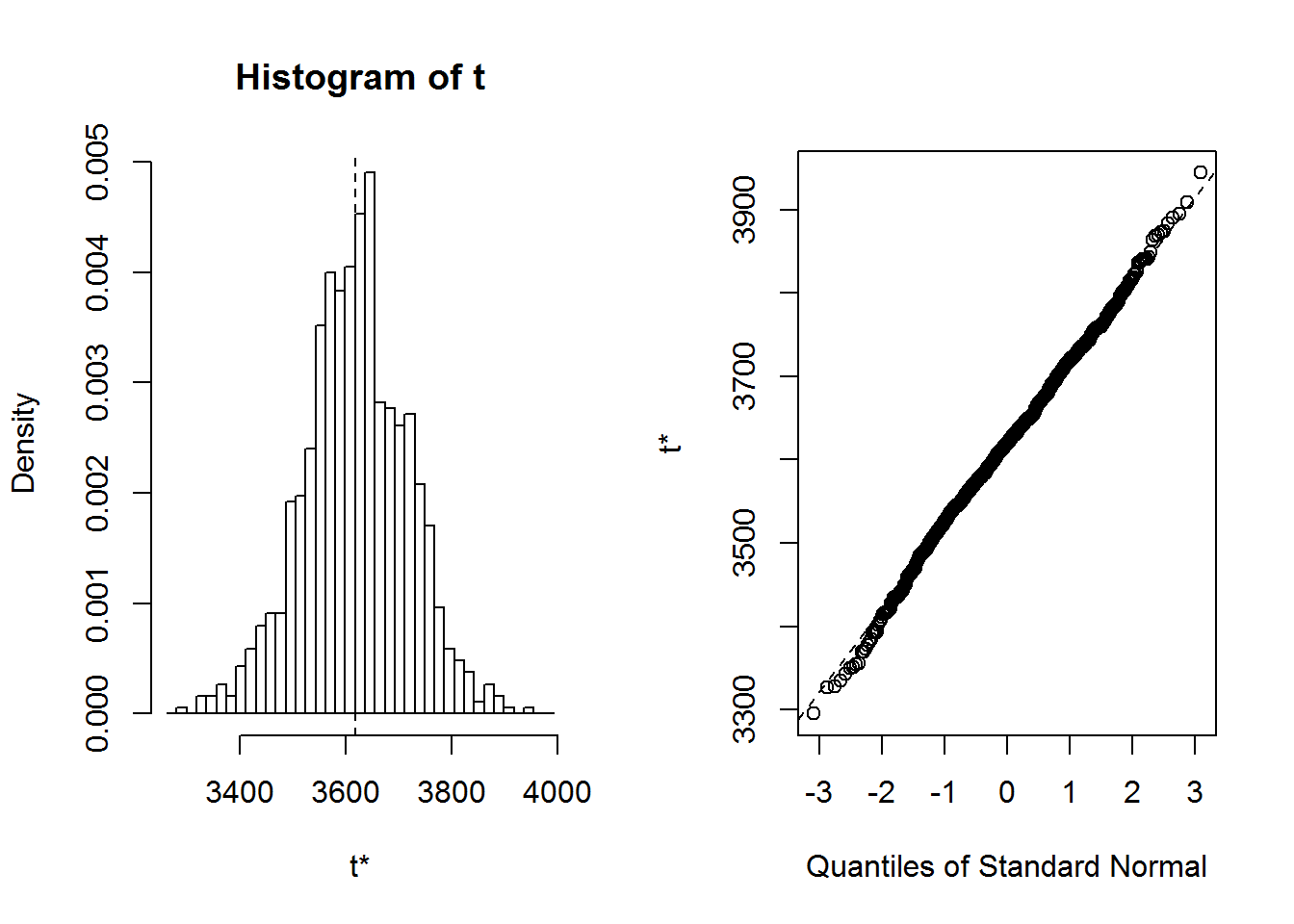Figure 4: Bootstrap sampling distribution of sample mean

Or, one can use ggplot to get the same figure

binw <- 2*(IQR(B$t)/length(B$t)^(1/3))
ggplot(data.frame(B$t), aes(B.t)) + geom_histogram(fill="grey", colour="black", binwidth = binw) + geom_vline(xintercept = B$t0, linetype="dashed", size=1) + labs(x="Bootstrap sample statistic", title="Bootstrap sampling distribution of sample mean")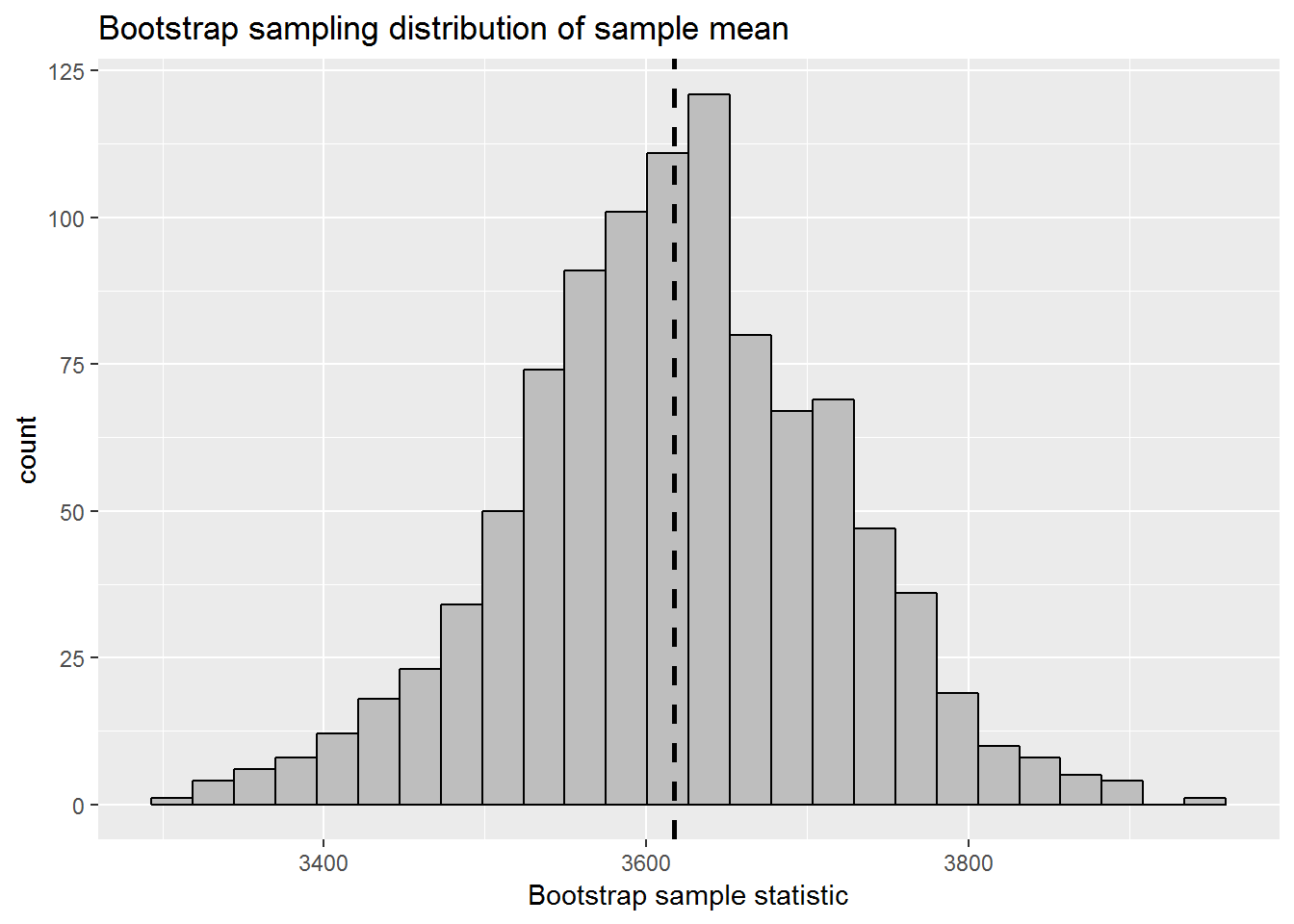Figure 5: Bootstrap sampling distribution of sample mean (ggplot2)

We can get confidence interval by,

boot.ci(B)
## BOOTSTRAP CONFIDENCE INTERVAL CALCULATIONS
## Based on 1000 bootstrap replicates
##
## CALL :
## boot.ci(boot.out = B)
##
## Intervals :
## Level      Normal              Basic
## 95%   (3423, 3810 )   (3421, 3819 )
##
## Level     Percentile            BCa
## 95%   (3416, 3814 )   (3407, 3804 )
## Calculations and Intervals on Original Scale

## Parametric Bootstrap

Once we have a sample data, we may think that the observed data may have come from some known probability distribution function ( i.e normal, gamma or poisson or any).

For example in our sample birth weights, we may assume that observed birth-weight comes from normal distribution with parameter $$\mu$$ = 3617.7 and $$\sigma$$ = 464.6 ( which is estimated from observed data). See the below figure,

ggplot(data.frame(df), aes(df)) + geom_density() + labs(x= "birth weight")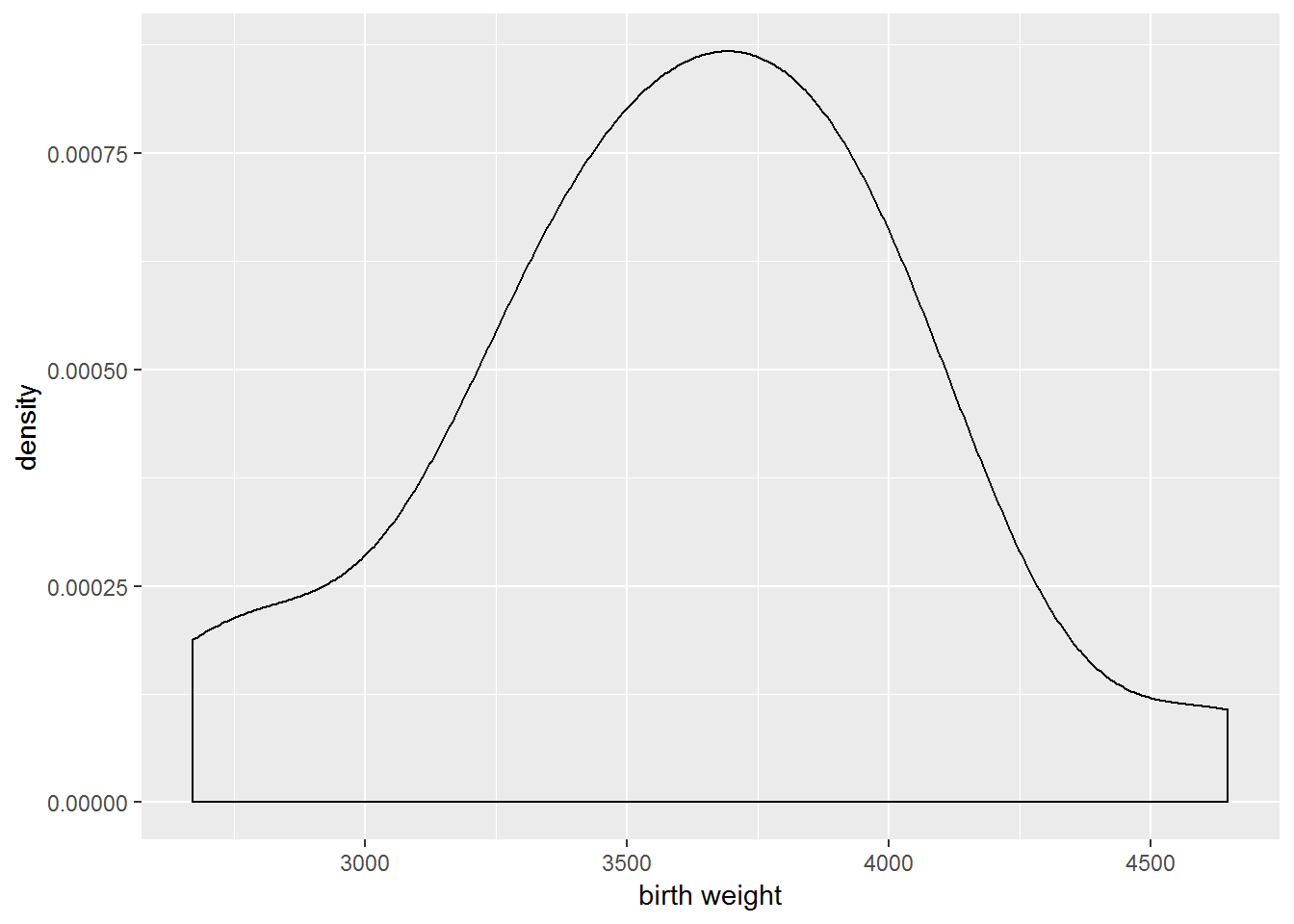Figure 6: Distribution of observed sample birthweights

So, instead of using observed data ( as a non-parametric bootstrap), we can use normal distribution function with probable parameter estimates ( which most likely the maximum likelihood estimates) for bootstrap re-sampling.

Let, first do it with help of for loops in R.

theta_star <- vector()
for (i in 1:1000){
theta_star[i] <- mean(rnorm(length(df),mean = 3617.7 ,sd = 464.6))
}
theta_boot <- mean(theta_star) # bootstrap estimate of theta_hat
theta_boot
##  3613.883
boot_se <- sd(theta_star) # standard eorrs of the estimate
boot_se
##  101.4233
bias <- theta_boot - mean(df)
bias
##  -3.769489
MSE <- mean((theta_boot- mean(df))^2)
MSE
##  14.20905
CI <-c(theta_boot-1.96*boot_se,theta_boot +1.96*boot_se)
CI
##  3415.094 3812.673

Now, using boot function,

For parametric bootstrap, one has to specify a function in ran.gen arguments, which tell the boots how random sample will be generated ( I mean, from which distribution, parameters you want to generate re-sample). The first argument will be the observed data and the second argument will be parameter estimates.This function should give random samples according to your assumed distribution function.

gen_function <- function(x,mle) {
data <- rnorm(length(x),mle,mle)
return(data)}
# function to calculate sample statistic
theta_star_function <- function(x,i) mean(x[i])

The mle values ( of parameter estimates ) passed to random generator function.

B <- boot(data = df, sim = "parametric", ran.gen =  gen_function, mle = c(3617.7,464.6), statistic = theta_star_function, R=1000)
B
##
## PARAMETRIC BOOTSTRAP
##
##
## Call:
## boot(data = df, statistic = theta_star_function, R = 1000, sim = "parametric",
##     ran.gen = gen_function, mle = c(3617.7, 464.6))
##
##
## Bootstrap Statistics :
##     original     bias    std. error
## t1* 3617.653 0.05774845    101.8872
plot(B)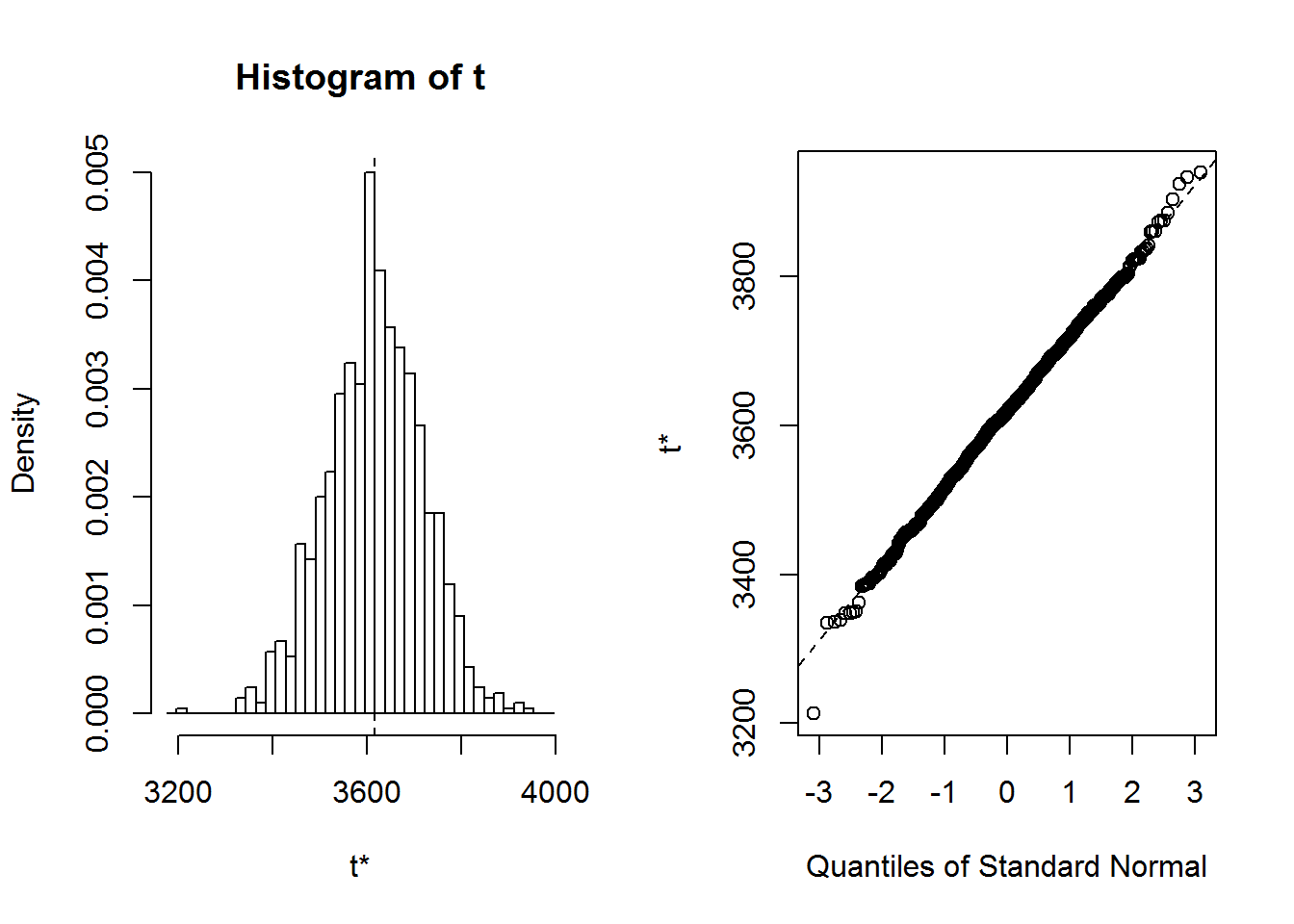Figure 7: Parametric bootstrap using boot package

boot.ci(B,type = "perc")
## BOOTSTRAP CONFIDENCE INTERVAL CALCULATIONS
## Based on 1000 bootstrap replicates
##
## CALL :
## boot.ci(boot.out = B, type = "perc")
##
## Intervals :
## Level     Percentile
## 95%   (3413, 3813 )
## Calculations and Intervals on Original Scale